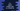# C++ program to delete the middle node in a linked list## C++ program to delete the middle node in a linked list:

Deleting the middle node requires the length. If we know the length, finding out the middle node is easy. For an even list, (length/2 + 1) is the middle node and for an odd list, (length + 1)/2 is the middle node position. For a linked list, we can have an infinite number of nodes and finding the length requires traversing the list. But there is a way that can be used to find the mid node in a linked list. In this post, I will show you how to find the middle node and how to delete it in a linked list.

### Finding the middle node in a linked list:

As I mentioned above, finding out the middle node is the tricky part. For that we will use the following steps :

1. Create two node pointers both pointed to head at start.
2. Move one pointer by one step and move another by two steps.
3. Repeat step 2 until the faster pointer reaches the end of the linked list.
4. If the fast pointer reaches the end, the another pointer will reach the middle. We can delete this middle node.

Let’s check the C++ program to write these steps:

### C++ program to delete the middle node in a linked list:

Below is the complete C++ program:

``````#include <iostream>
using namespace std;

struct Node
{
int value;
Node *next;
};

{

public:
{
Node *node = new Node;
node->value = value;

{
node->next = NULL;
}
else
{
}
}

void deleteMiddle()
{
{
return;
}

while (fastPointer && fastPointer->next && fastPointer->next->next)
{
slowPointer = slowPointer->next;
fastPointer = fastPointer->next->next;
}

slowPointer->next = slowPointer->next->next;
}

void displayList()
{
<< "->";
while (flag != NULL)
{
cout << flag->value << "->";
flag = flag->next;
}
cout << "NULL" << endl;
}
};

int main()
{
int total, value;

cout << "Enter the total nodes :" << endl;
cin >> total;

for (int i = 0; i < total; i++)
{
cout << "Enter value for node " << i << " :" << endl;
cin >> value;
}

cout << "Printing the linked list :" << endl;
cout << "After middle node is deleted :"<<endl;
return 0;
}``````

### Explanation:

1. Node structure is used to define a node of the linked list. It can hold one integer value and pointer to the next node.
2. LinkedList class is to handle all linked list operations. This class has three public methods. displayList to display the full list, insertAtHead to insert one node at head,and deleteMiddle to delete the middle node.
4. In the deleteMiddle method, we are defining two pointers at first : slowPointer and fastPointer. At start, slowPointer is pointing to the first node and fastPointer pointing to the second node.
5. Using a while loop, we are iterating through the linked list. On each step, slowPointer is moving one node and fastPointer is moving two nodes.
6. Once the while loop ends, then we are removing the mid node by pointing its previous node to its next node.

### Sample output:

If you execute the above program, it will give output as like below:

``````Enter the total nodes :
5
Enter value for node 0 :
1
Enter value for node 1 :
2
Enter value for node 2 :
3
Enter value for node 3 :
4
Enter value for node 4 :
5
After middle node is deleted :

Enter the total nodes :
4
Enter value for node 0 :
1
Enter value for node 1 :
2
Enter value for node 2 :
3
Enter value for node 3 :
4
After middle node is deleted :